2020-02-26 17:32:29

## 一行Python代码能实现什么奇葩功能？

17 / 0 / 0 / 0

mandelbrot 图像中文名叫曼德勃罗图像。Mandelbrot 图像中的每个位置都对应于公式 N=x+y*i 中的一个复数。其实数部分是 x，虚数部分是 y，i 是 -1 的平方根。图像中各个位置的 x 和 y 坐标对应于虚数的 x 和 y 部分。百度百科给出的图形是这样的。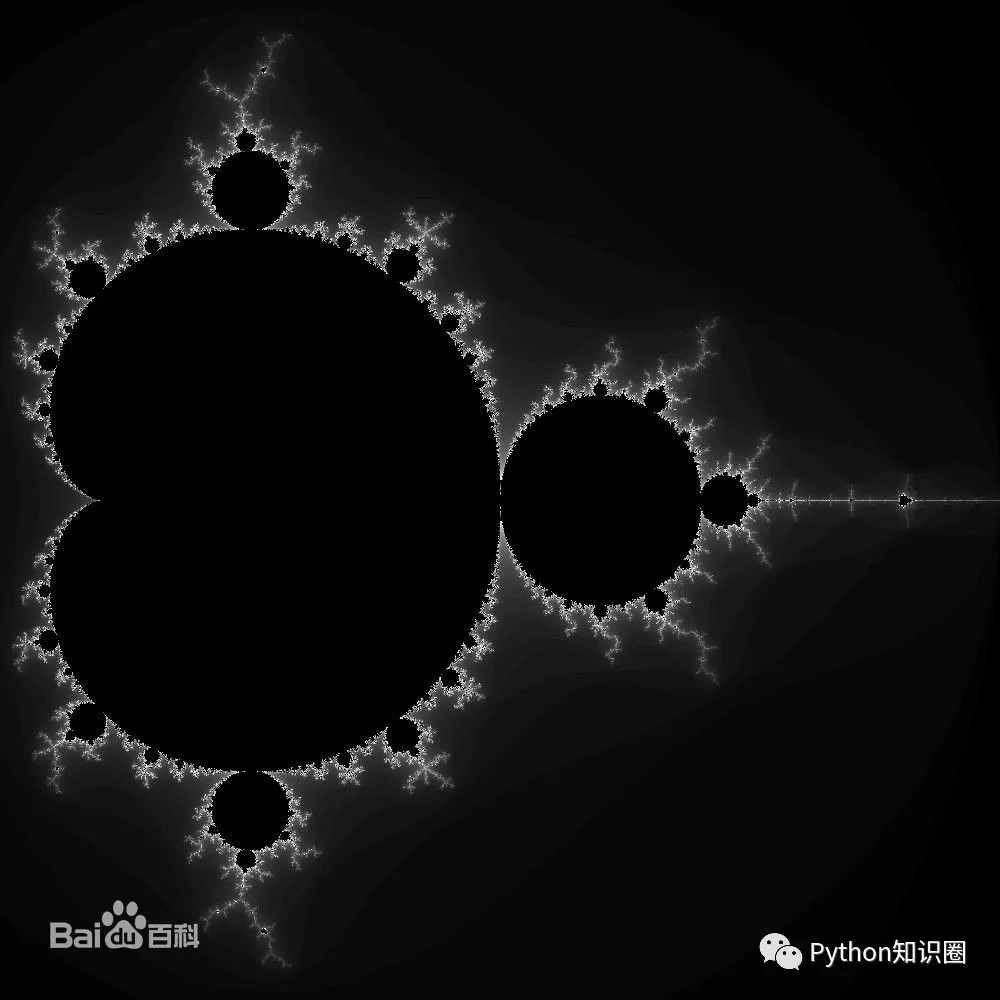print('\n'.join([''.join(['*'if abs((lambda a: lambda z, c, n: a(a, z, c, n))(lambda s, z, c, n: z if n == 0 else s(s, z*z+c, c, n-1))(0, 0.02*x+0.05j*y, 40)) < 2 else ' ' for x in range(-80, 20)]) for y in range(-20, 20)]))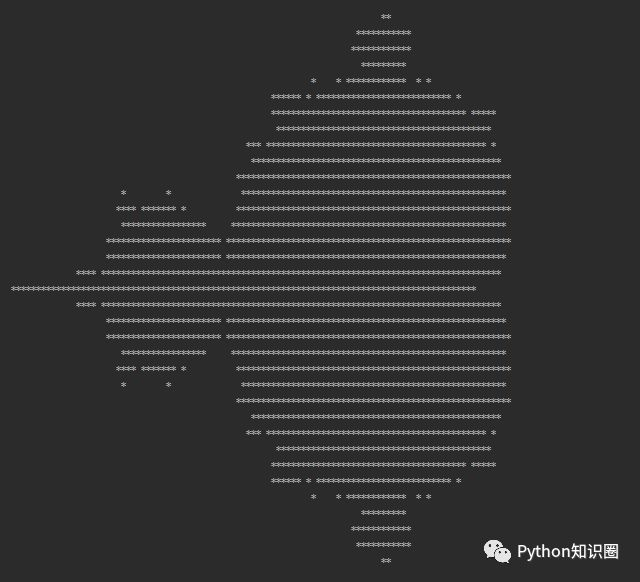print('\n'.join([' '.join(['%s*%s=%-2s' % (y, x, x*y) for y in range(1, x+1)]) for x in range(1, 10)]))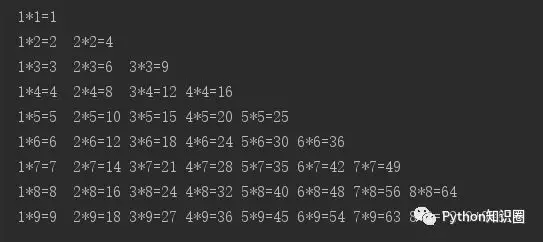print('\n'.join([''.join([('AndyLove'[(x-y)%8]if((x*0.05)**2+(y*0.1)**2-1)**3-(x*0.05)**2*(y*0.1)**3<=0 else' ')for x in range(-30,30)])for y in range(15,-15,-1)]))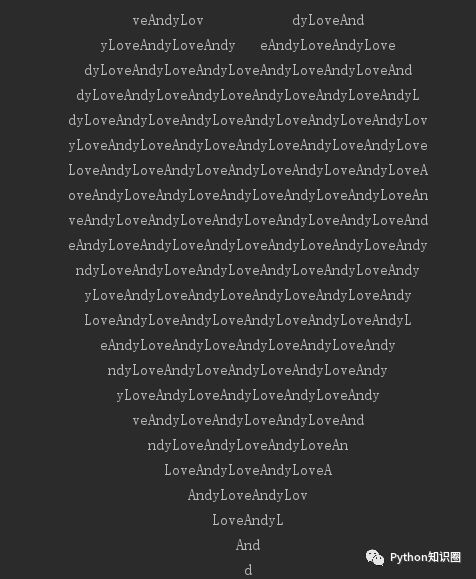exec("""\nfrom turtle import *\nfor i in range(500): \n    forward(i)\n    left(91)\n""")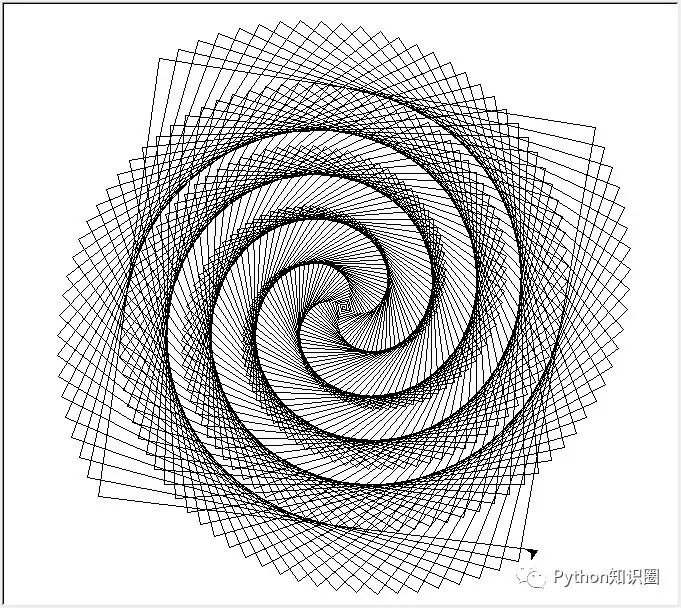print(' '.join([str(item) for item in filter(lambda x: not [x % i for i in range(2, x) if x % i == 0], range(2, 101))]))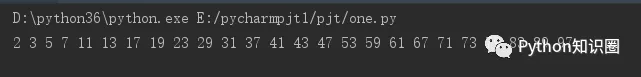print([x for x in [(a[i], a.append([a[i], a[i]+a[i]])) for a in ([[1, 1]], ) for i in range(30)]])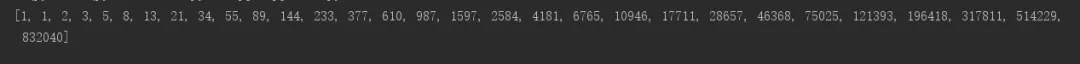quickSort = lambda array: array if len(array) <= 1 else quickSort([item for item in array[1:] if item <= array]) + [array] + quickSort([item for item in array[1:] if item > array])
array = [9, 11, 88, 32, 8]
print(quickSort(array))

>>> import this
The Zen of Python, by Tim Peters

Beautiful is better than ugly.
Explicit is better than implicit.
Simple is better than complex.
Complex is better than complicated.
Flat is better than nested.
Sparse is better than dense.
Special cases aren't special enough to break the rules.
Although practicality beats purity.
Errors should never pass silently.
Unless explicitly silenced.
In the face of ambiguity, refuse the temptation to guess.
There should be one-- and preferably only one --obvious way to do it.
Although that way may not be obvious at first unless you're Dutch.
Now is better than never.
Although never is often better than *right* now.
If the implementation is hard to explain, it's a bad idea.
If the implementation is easy to explain, it may be a good idea.
Namespaces are one honking great idea -- let's do more of those!

import antigravity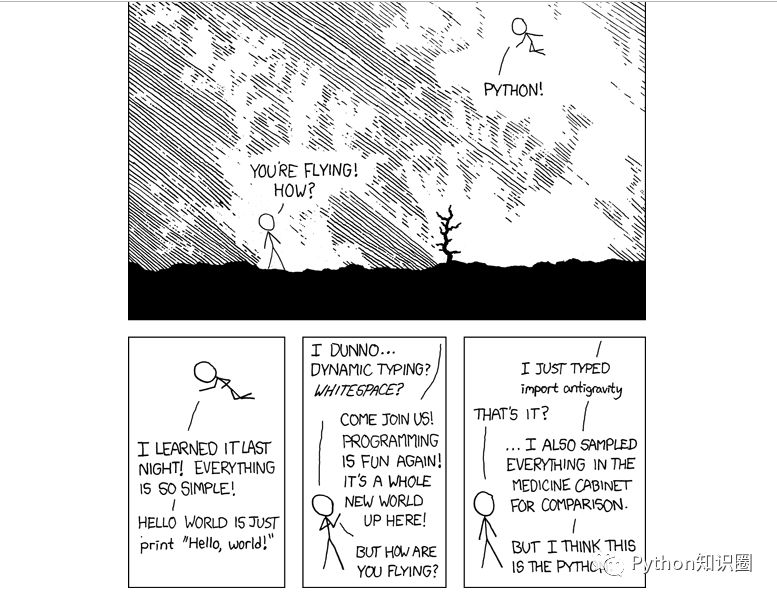“你在飞！怎么做到的？”
“Python!”

“我昨晚学习了 Python，一切都是那么简单”

“运行 HELLO WORLD 只需要 print “Hello World!””

“我还是不明白……动态类型，还是空格？”

“来加入我们吧，编程又再次变得有趣起来了，Python 是一个全新的世界”

“但你是怎么飞起来的？”

“我只是输入了 import antigravity”

“就这样？”

“我还对药品柜中的所有东西进行了采样比较”（暗指他对比过多种编程语言，但还是觉得 Python 最简单）

“但我想这就是 Python.”

cmd 命令下输入下列代码实现单线迷宫。

python -c "while 1:import random;print(random.choice('|| __'), end='')"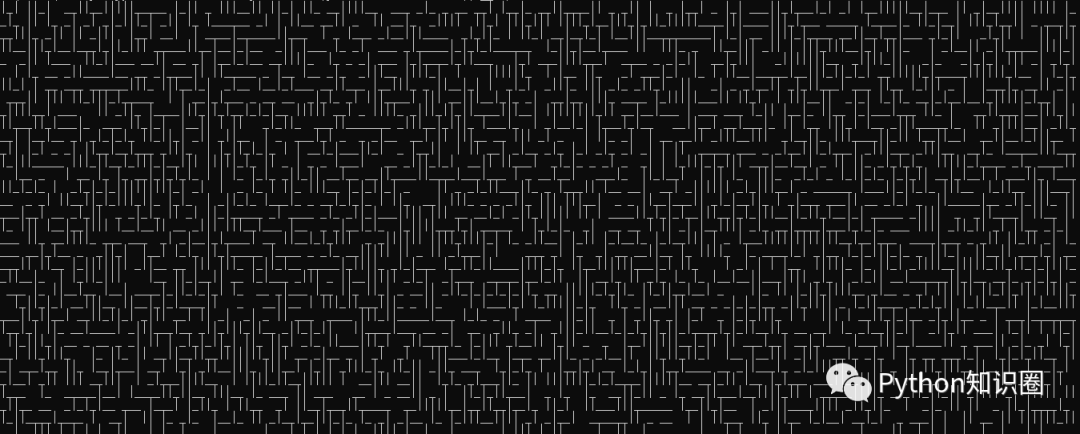python -m http.server 8080python -c "import random;p=lambda:random.choice('7♪♫♣♠♦♥◄☼☽');[print('|'.join([p(),p(),p()]),end='\r') for i in range(8**5)]”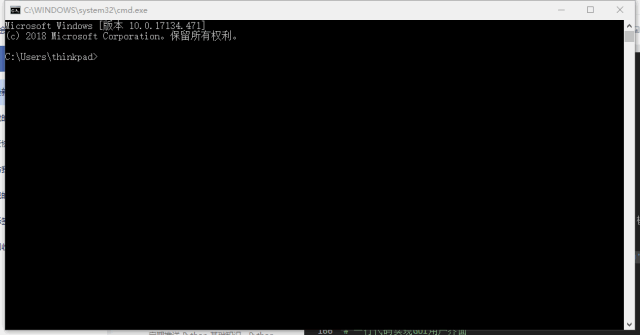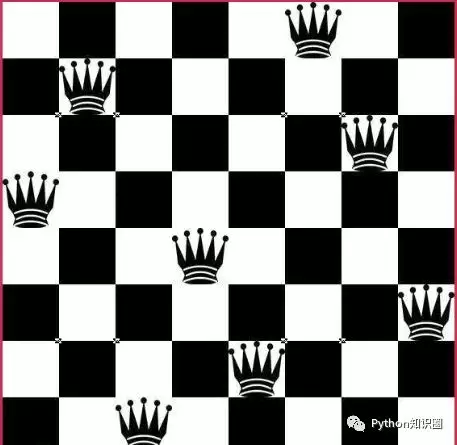[__import__('sys').stdout.write('\n'.join('.' * i + 'Q' + '.' * (8-i-1) for i in vec) + "\n========\n") for vec in __import__('itertools').permutations(range(8)) if 8 == len(set(vec[i]+i for i in range(8))) == len(set(vec[i]-i for i in range(8)))]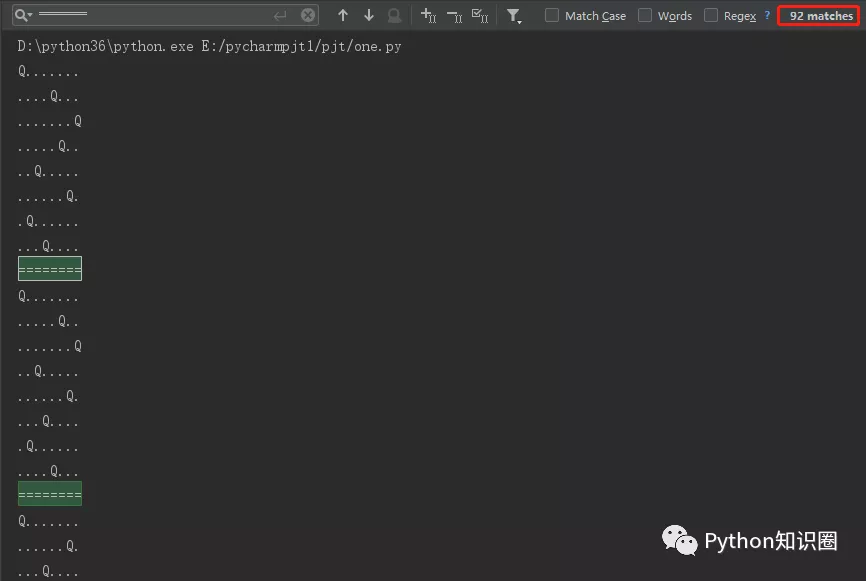print([i for item in ab for i in item])  

ab = [[1, 2, 3], [5, 8], [7, 8, 9]]
print([i for item in ab for i in item])

# 结果
[1, 2, 3, 5, 8, 7, 8, 9]

2 的 1000 次方的值很大，把结果每位数都加起来等于多少呢？

print(sum(map(int, str(2**1000))))

# 结果
1366

print("I Love You!\n" * 3000)

PS: 如本文对您有疑惑，可加QQ：1752338621 进行讨论。

0
0